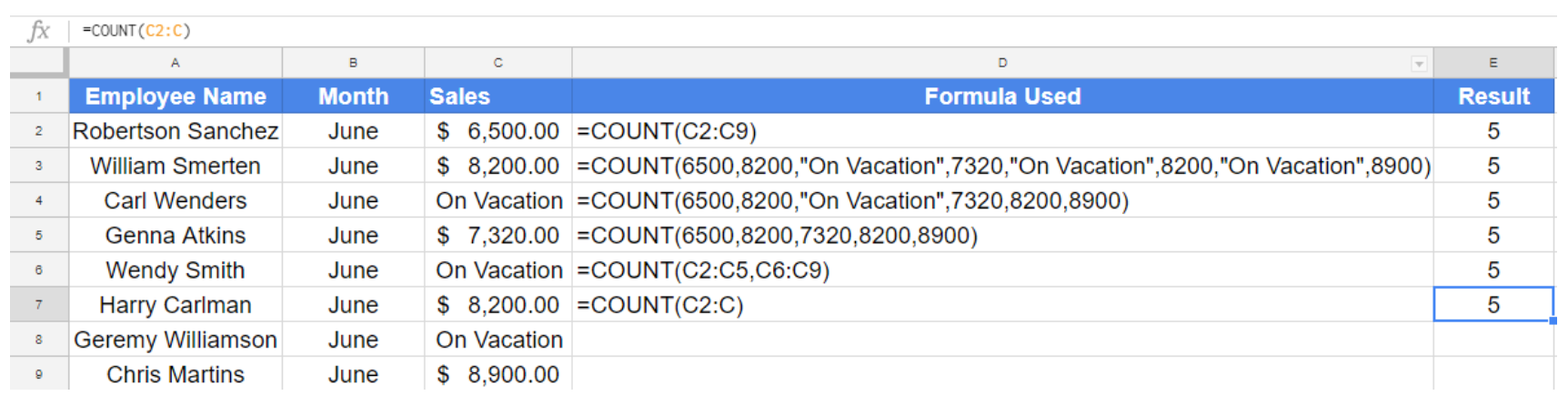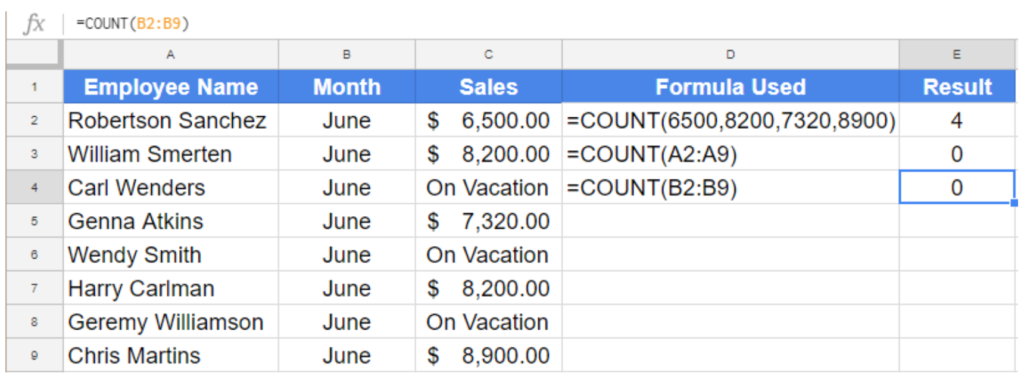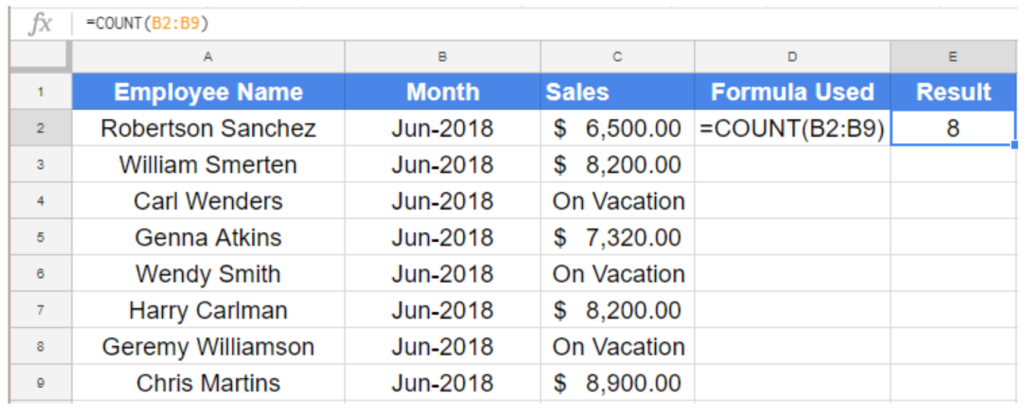Get instant live expert help with Excel or Google Sheets“My Excelchat expert helped me in less than 20 minutes, saving me what would have been 5 hours of work!”

#### Post your problem and you’ll get expert help in seconds.

Your message must be at least 40 characters
Our professional experts are available now. Your privacy is guaranteed.

# How to Use the Count Function in Google Sheets

The COUNT formula in Google Sheets extracts the number of time a numeric value exists in a range. COUNT is one of the simplest and most used formulas in Google Sheets. In this tutorial, you will learn how to use the COUNT function in Google Sheets.

## Using the COUNT function in Google Sheets

### Syntax using COUNT

The COUNT function in Google Sheets follows the syntax

`=COUNT(value1,[value2],…)`

### Arguments

value1: Required. The value or the reference to a range which you want to count inside.

value2: Optional. Values or ranges, in addition, to count numbers within. You can add up to 255 additional values or ranges.

### Notes

• You can use the value itself, cell reference, named range, cell ranges as an argument.

• The Google Sheets COUNT function can count numbers, formulas, logical expressions (TRUE/FALSE) and dates.

• If you modify the content of the range used as an argument to a COUNT function, the formula recalculates the result automatically.

• If a value repeats in multiple cells that are used as an argument to a COUNT function, COUNT calculates each time regardless of the repetition.

### Applying COUNT function in Google Sheets examples

The following examples contain sales data for different employees. It includes the employee names, month and sales for the month of June.Column D contains the formulas used, and Column E has the results for the corresponding formulas. The six formulas in use here are:

In cell E1:

`=COUNT(C2:C9)`

Counts the numeric values between cells C2 to C9. Returns the number 5 and ignores the text value On Vacation in cells C4, C6 and C8. If we write C2:C9 explicitly, the formula will be as in cell E2:

`=COUNT(6500,8200,"On Vacation",7320,"On Vacation",8200,"On Vacation",8900)`

Since COUNT doesn’t take text values, the formula above equals to counts the numbers 6500,8200, 7320 and 8900. Counts 8200 twice though it is a repeated value. In cell E3, although the text value “On Vacation” is included in the formula, COUNT ignores it, see below.

`=COUNT(6500,8200,"On Vacation",7320,8200,8900)`

In cell E4, the formula works in the same way as the previous one. The following formula counts the numbers provided in the arguments and ignores the text value.

`=COUNT(6500,8200,7320,8200,8900)`

Cell E5 counts the numeric values provided in the argument:

`=COUNT(C2:C5,C6:C9)`

This formula counts the values from cells C2 to C5 and C6 to C9. It counts the numeric values ignoring the repeated values and text values.

`=COUNT(C2:C)`

Cell E6 counts the number value in cell C2 and all other cells after C2 in column C. This is an excellent way to count numbers in an entire column if you are going to add data in the future.

### COUNT function and data format

All of the above-mentioned formulas return the number 5. However, that is not always the case. COUNT function in Google Sheets recognizes number and date but will not recognize text. Let us see some cases where COUNT returns different results from normal expectation.`=COUNT(6500,8200,7320,8900)`

Counts the number of instants for the numbers provided in the arguments. Despite the number 8200 being repeated in cells C3 and C7, the COUNT formula in google sheets counts it once and returns the number 4.

`=COUNT(A2:A9)`

Counts the numeric values between cell A2 and A9. Eventually returns 0 as the values are all text.

`=COUNT(B2:B9)`

Counts the number values from B2 to B9. Returns 0 like the previous example as the values are not numeric. However, if the value were formatted as dates, Google Sheets COUNT function would have counted them.In this example, the months are formatted as dates. Therefore, the formula `=COUNT(B2:B9)` now returns the value 8.

Still need some help with Excel formatting or have other questions about Excel? Connect with a live Excel expert here for some 1 on 1 help. Your first session is always free.

### Did this post not answer your question? Get a solution from connecting with the expert.Another blog reader asked this question today on Excelchat:
Solution examplesUse the Vlookup Function to complete the "employee" column of table 2. Use "job Id" from table 2 as your lookup_value(s) and table 1 as your reference.
Solved by C. H. in 16 minsIf a cell in another sheet is populated I need a vlookup done. If the cell is not populated I need the cell to return blank.
Solved by T. D. in 60 minsI am trying to make a chart that turns a week range red if nothing is entered in the range. If something is entered then I would like it to turn green. Please Help
Solved by E. U. in 43 minsI need a check box to show/hide an answer of an if function
Solved by Z. U. in 23 minsI need a formula to compare the data in two columns and then export the mismatched data in the 3rd column
Solved by S. Q. in 20 mins## Subscribe to Excelchat.coAnother blog reader asked this question today on Excelchat: